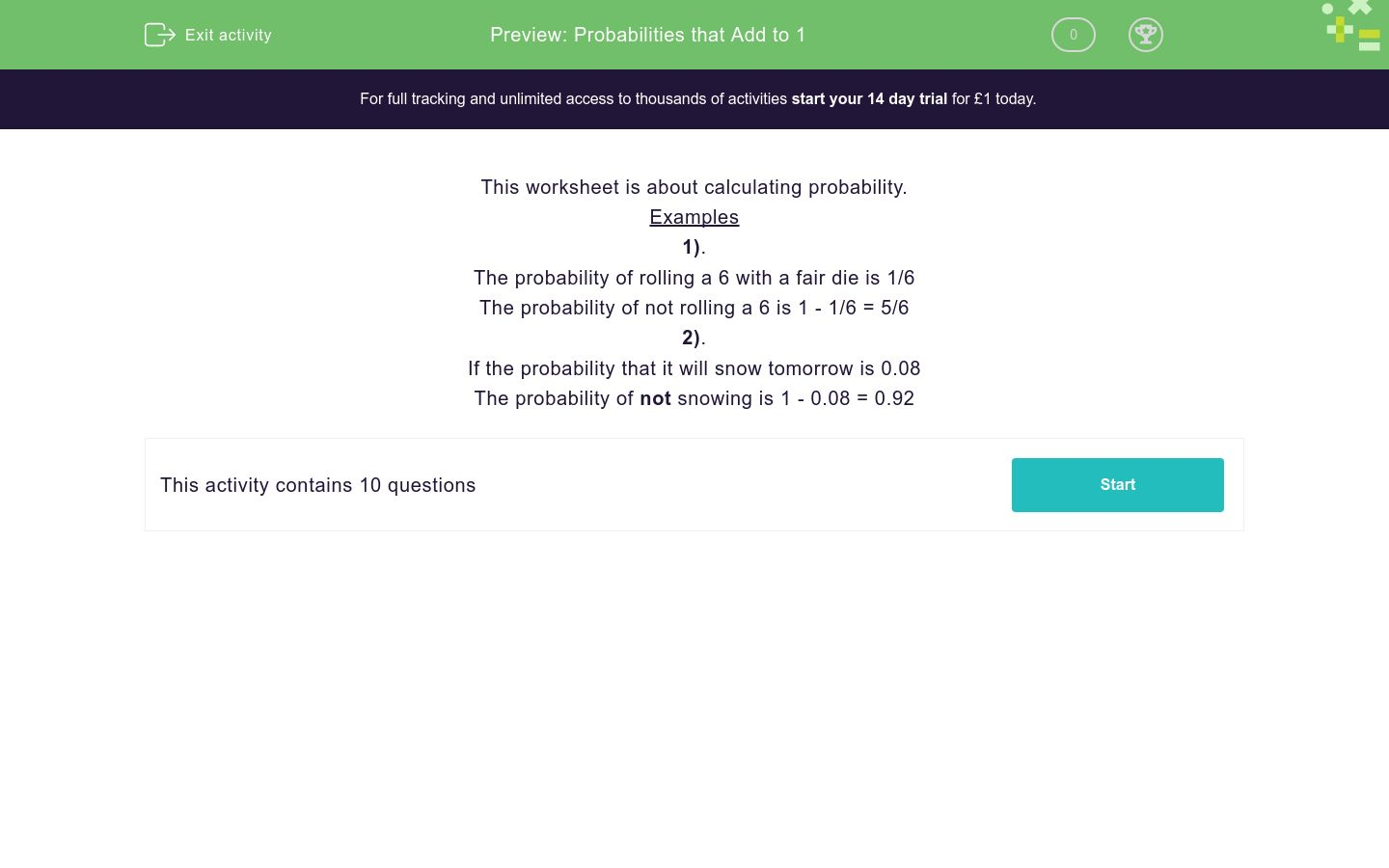# Probabilities that Add to 1

In this worksheet, students calculate probabilities.Key stage:  KS 4

Curriculum topic:  Probability

Difficulty level:### QUESTION 1 of 10

This worksheet is about calculating probability.

Examples

1).

The probability of rolling a 6 with a fair die is 1/6

The probability of not rolling a 6 is 1 - 1/6 = 5/6

2).

If the probability that it will snow tomorrow is 0.08

The probability of not snowing is 1 - 0.08 = 0.92

 A drawer contains 20 black, 16 brown, 20 blue, and 8 white socks. A sock is selected at random. What is the probability of getting

a black sock _____ ?

(write the fraction in its lowest terms)

 A drawer contains 20 black, 16 brown, 20 blue, and 8 white socks. A sock is selected at random. What is the probability of getting

a brown OR a blue sock _____ ?

 A drawer contains 20 black, 16 brown, 20 blue, and 8 white socks. A sock is selected at random. What is the probability of NOT getting

a blue sock _____ ?

In the word MISSISSIPPI a letter is choosen at random.

What is the probability of NOT choosing:

a letter M _____ ?

In the word MISSISSIPPI a letter is choosen at random.

What is the probability of not choosing:

a letter S _____ ?

In the word MISSISSIPPI a letter is choosen at random.

What is the probability of choosing:

M OR P _____ ?

A box of chocolates contains 21 dark chocolates, 19 milk, and 25 white.

A chocolate is choosen at random.

What is the probability that it is

NOT milk _____ ?

A box of chocolates contains 21 dark chocolates, 19 milk, and 25 white.

A chocolate is choosen at random.

What is the probability that it is

milk OR white _____ ?

A box of chocolates contains 21 dark chocolates, 19 milk, and 25 white.

A chocolate is choosen at random.

What is the probability that it is

dark OR white _____?

A box of chocolates contains 21 dark chocolates, 19 milk, and 25 white.

A chocolate is choosen at random.

What is the probability that it is

neither dark NOR white _____?

• Question 1
 A drawer contains 20 black, 16 brown, 20 blue, and 8 white socks. A sock is selected at random. What is the probability of getting

a black sock _____ ?

(write the fraction in its lowest terms)

CORRECT ANSWER
5/16
• Question 2
 A drawer contains 20 black, 16 brown, 20 blue, and 8 white socks. A sock is selected at random. What is the probability of getting

a brown OR a blue sock _____ ?

CORRECT ANSWER
9/16
• Question 3
 A drawer contains 20 black, 16 brown, 20 blue, and 8 white socks. A sock is selected at random. What is the probability of NOT getting

a blue sock _____ ?

CORRECT ANSWER
11/16
• Question 4

In the word MISSISSIPPI a letter is choosen at random.

What is the probability of NOT choosing:

a letter M _____ ?

CORRECT ANSWER
10/11
• Question 5

In the word MISSISSIPPI a letter is choosen at random.

What is the probability of not choosing:

a letter S _____ ?

CORRECT ANSWER
7/11
• Question 6

In the word MISSISSIPPI a letter is choosen at random.

What is the probability of choosing:

M OR P _____ ?

CORRECT ANSWER
3/11
• Question 7

A box of chocolates contains 21 dark chocolates, 19 milk, and 25 white.

A chocolate is choosen at random.

What is the probability that it is

NOT milk _____ ?

CORRECT ANSWER
46/65
• Question 8

A box of chocolates contains 21 dark chocolates, 19 milk, and 25 white.

A chocolate is choosen at random.

What is the probability that it is

milk OR white _____ ?

CORRECT ANSWER
44/65
• Question 9

A box of chocolates contains 21 dark chocolates, 19 milk, and 25 white.

A chocolate is choosen at random.

What is the probability that it is

dark OR white _____?

CORRECT ANSWER
46/65
• Question 10

A box of chocolates contains 21 dark chocolates, 19 milk, and 25 white.

A chocolate is choosen at random.

What is the probability that it is

neither dark NOR white _____?

CORRECT ANSWER
19/65
---- OR ----

Sign up for a £1 trial so you can track and measure your child's progress on this activity.

### What is EdPlace?

We're your National Curriculum aligned online education content provider helping each child succeed in English, maths and science from year 1 to GCSE. With an EdPlace account you’ll be able to track and measure progress, helping each child achieve their best. We build confidence and attainment by personalising each child’s learning at a level that suits them.

Start your £1 trial